Multiplication of matrices

Chapter 3 Class 12 Matrices
Concept wise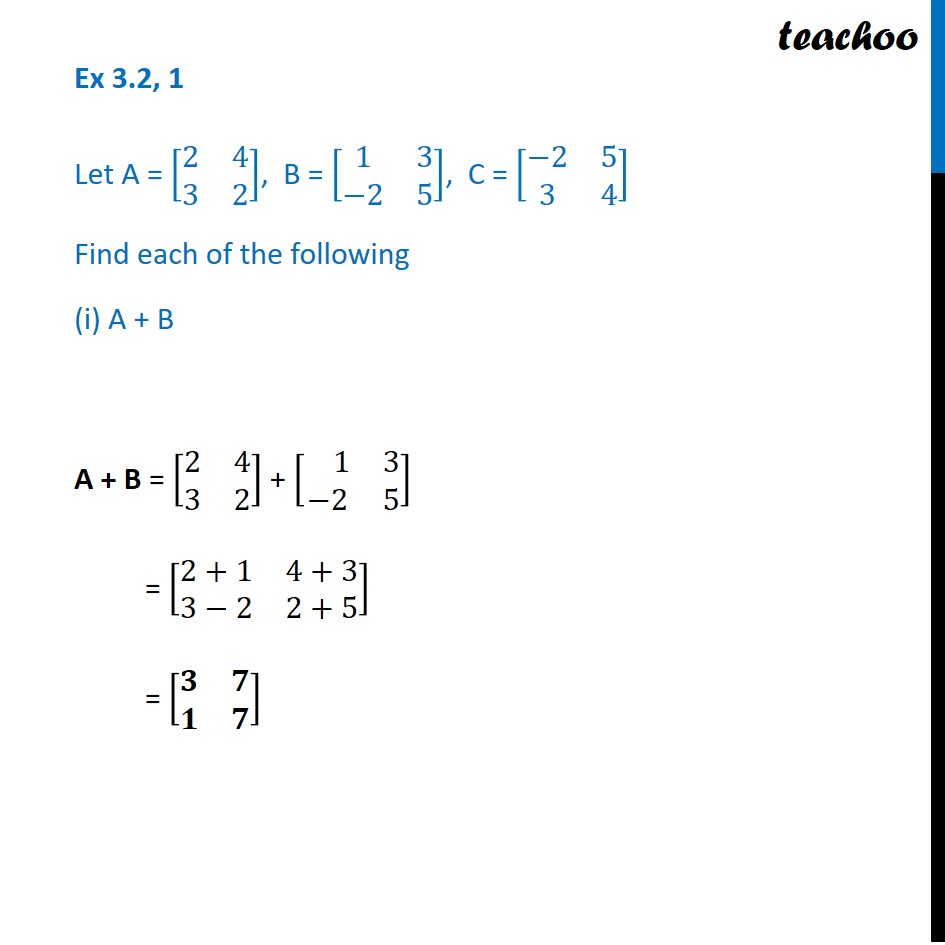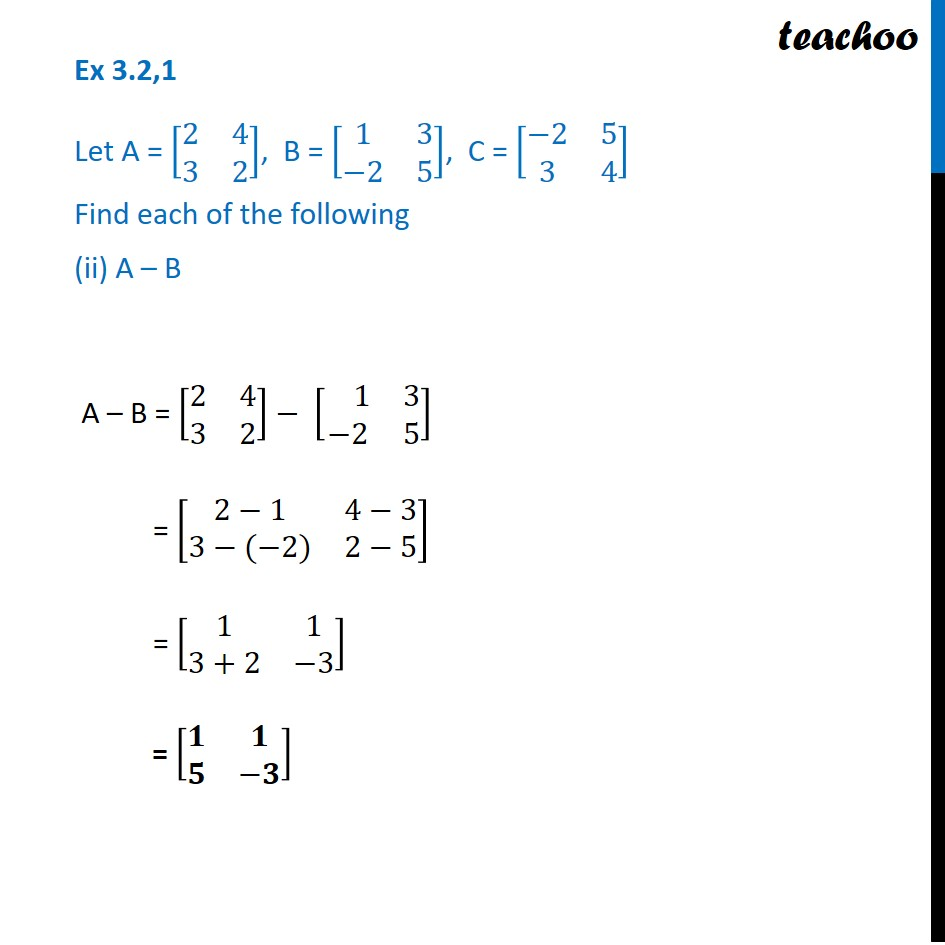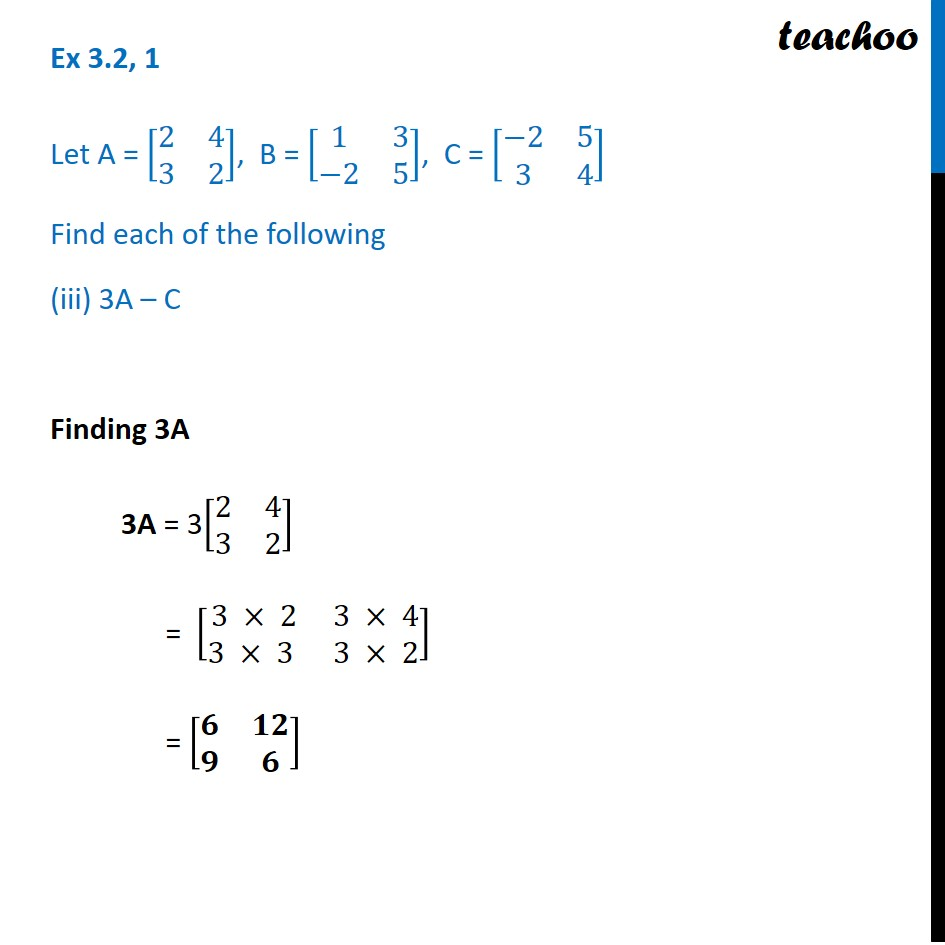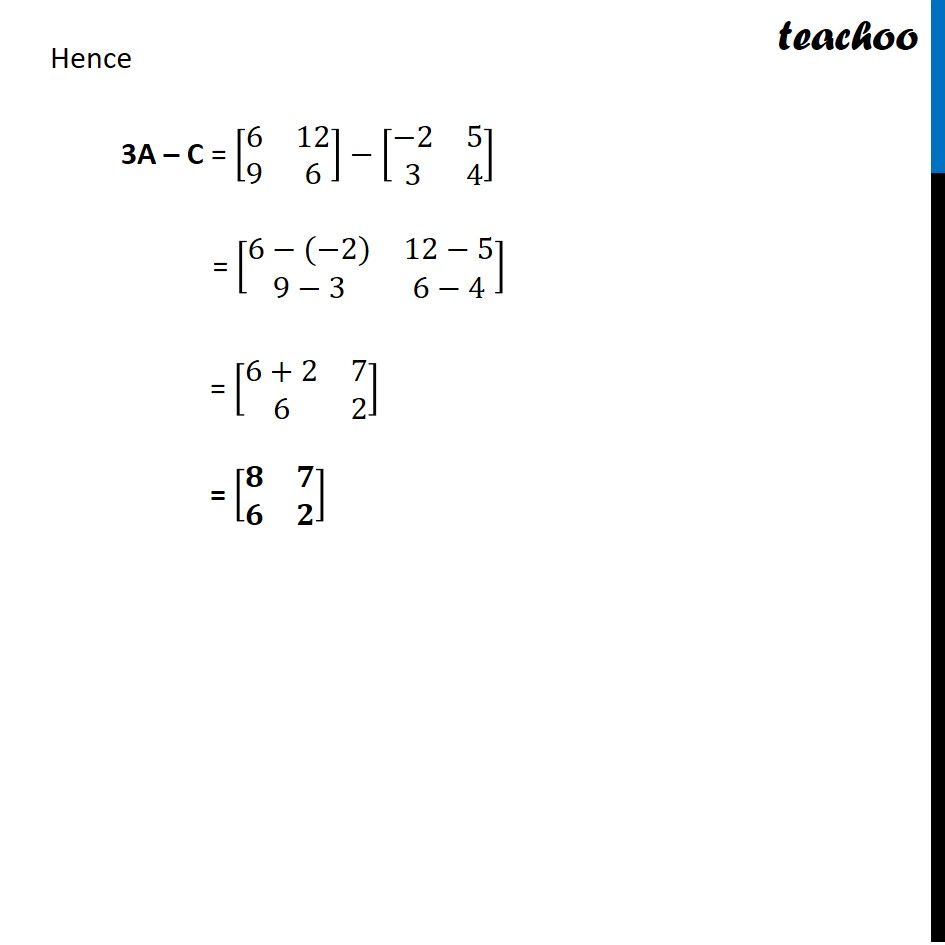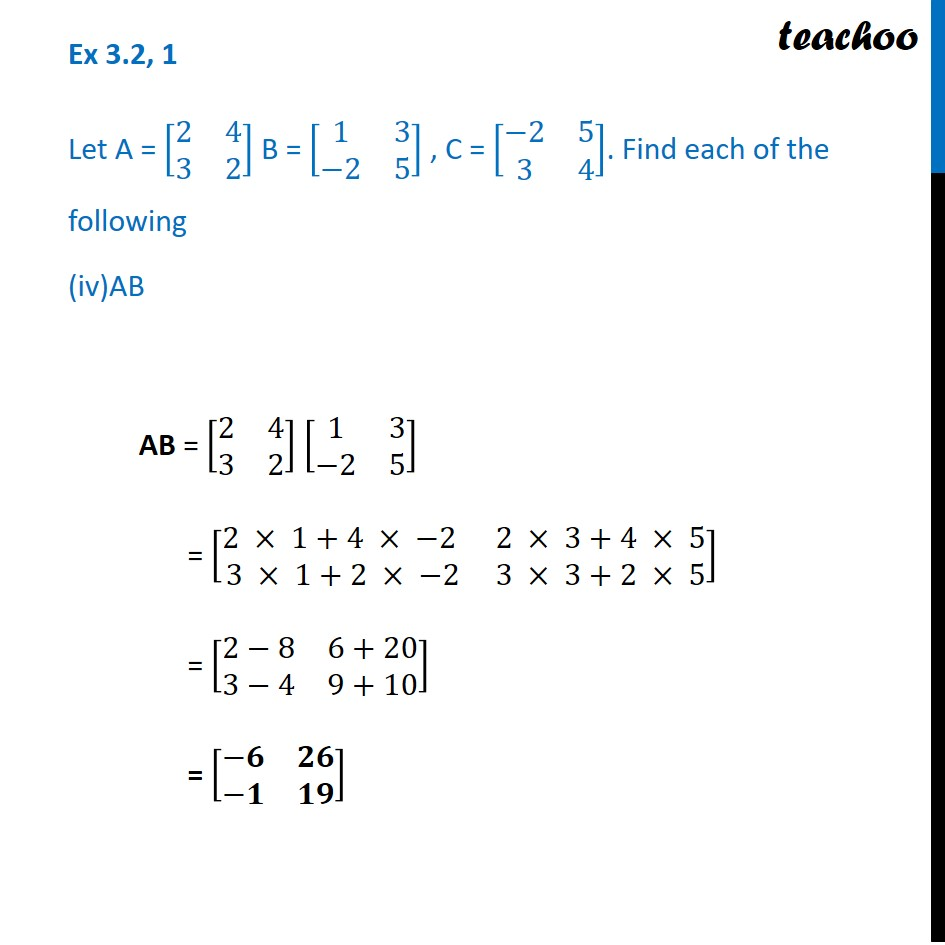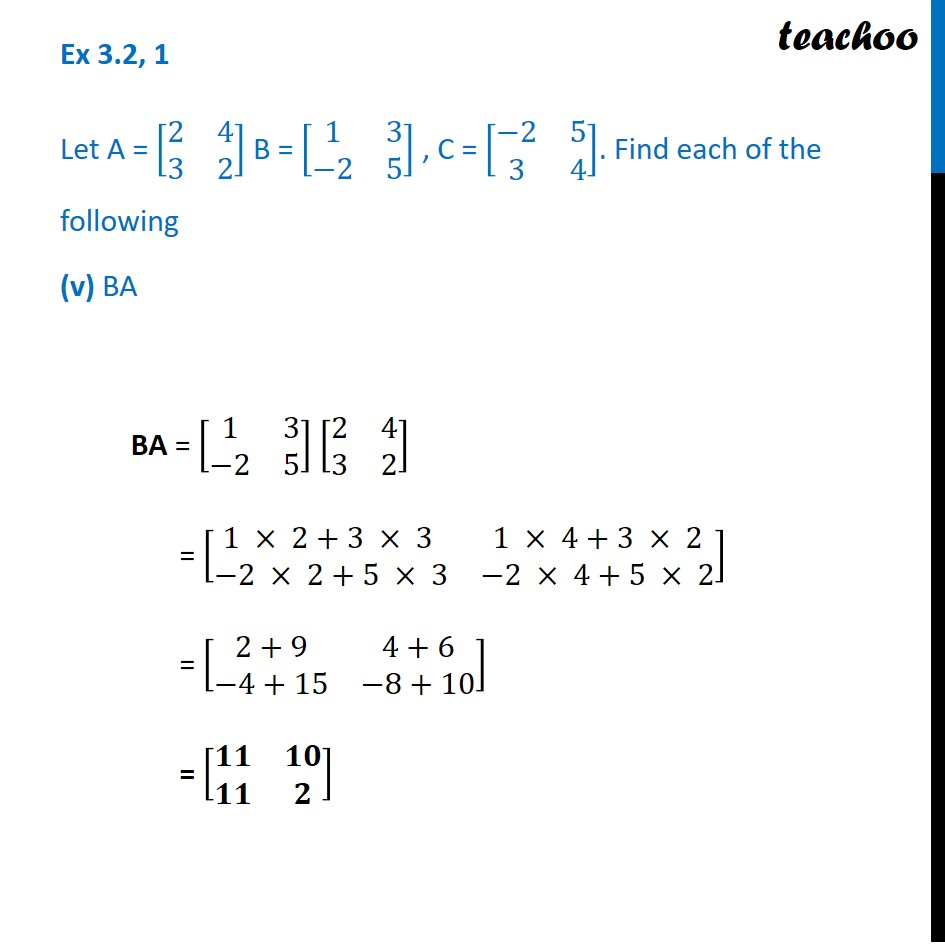Learn in your speed, with individual attention - Teachoo Maths 1-on-1 Class

### Transcript

Ex 3.2, 1 Let A = [■8(2&[email protected]&2)], B = [■8(1&3@−2&5)], C = [■8(−2&[email protected]&4)] Find each of the following (i) A + B A + B = [■8(2&[email protected]&2)] + [■8( 1&3@−2&5)] = [■8(2+1&[email protected]−2&2+5)] = [■8(𝟑&𝟕@𝟏&𝟕)] Ex 3.2,1 Let A = [■8(2&[email protected]&2)], B = [■8(1&3@−2&5)], C = [■8(−2&[email protected]&4)] Find each of the following (ii) A – B A – B = [■8(2&[email protected]&2)]− [■8( 1&3@−2&5)] = [■8(2−1&4−[email protected]−(−2)&2−5)] = [■8(1&[email protected]+2&−3)] = [■8(𝟏&𝟏@𝟓&−𝟑)] Ex 3.2, 1 Let A = [■8(2&[email protected]&2)], B = [■8(1&3@−2&5)], C = [■8(−2&[email protected]&4)] Find each of the following 3A – C Finding 3A 3A = 3[■8(2&[email protected]&2)] = [■8(3 × 2&3 × [email protected] × 3 &3 × 2)] = [■8(𝟔&𝟏𝟐@𝟗&𝟔)] Hence 3A – C = [■8(6&[email protected]&6)] ⤶7− [■8(−2&[email protected]&4)] = [■8(6−(−2)&12−[email protected]−3&6−4)] = [■8(6+2&[email protected]&2)] = [■8(𝟖&𝟕@𝟔&𝟐)] Ex 3.2, 1 Let A = [■8(2&[email protected]&2)] B = [■8(1&3@−2&5)] , C = [■8(−2&[email protected]&4)]. Find each of the following (iv)AB AB = [■8(2&[email protected]&2)] [■8(1&3@−2&5)] = [■8(2 × 1+4 × −2 &2 × 3+4 × [email protected] × 1+2 × −2&3 × 3+2 × 5)] = [■8(2−8&[email protected]−4&9+10)] = [■8(−𝟔&𝟐𝟔@−𝟏&𝟏𝟗)] Ex 3.2, 1 Let A = [■8(2&[email protected]&2)] B = [■8(1&3@−2&5)] , C = [■8(−2&[email protected]&4)]. Find each of the following (v) BA BA = [■8(1&3@−2&5)] [■8(2&[email protected]&2)] = [■8(1 × 2+3 × 3 &1 × 4+3 × 2@−2 × 2+5 × 3&−2 × 4+5 × 2)] = [■8(2+9&4+6@−4+15&−8+10)] = [■8(𝟏𝟏&𝟏𝟎@𝟏𝟏&𝟐)]